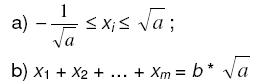Online JudgeProblem SetAuthorsOnline ContestsUser
Web Board
F.A.Qs
Statistical Charts
Problems
Submit Problem
Online Status
Prob.ID:
Register
Authors ranklist
Current Contest
Past Contests
Scheduled Contests
Award Contest
Register

Language:
Maximum
 Time Limit: 1000MS Memory Limit: 65536K Total Submissions: 919 Accepted: 539

Description

Let x1, x2, ..., xm be real numbers satisfying the following conditions:for some integers a and b (a > 0).

Determine the maximum value of x1p + x2p + … + xmp for some even positive integer p.

Input

Each input line contains four integers: m, p, a, b (m <= 2000, p <= 12, p is even). Input is correct, i.e. for each input numbers there exists x1, x2, …, xm satisfying the given conditions.

Output

For each input line print one number – the maximum value of expression, given above. The answer must be rounded to the nearest integer.

Sample Input

```1997 12 3 -318
10 2 4 -1```

Sample Output

```189548
6```

Source

[Submit]   [Go Back]   [Status]   [Discuss]Home PageGo BackTo top